1 详细解释imageTtfText()函数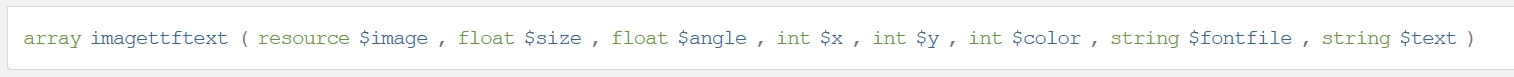（1）\$image 这个是画布资源，无需再解释；
（2）\$size，官方文档的解释是，字体大小，其长度单位依赖于GD库的版本，对于GD1来说是像素，对于GD2来说是磅（point)。现在一般都是GD2了，那么这个磅究竟是什么意思呢？这涉及到字体设计的基本知识。

1磅 = PPI/72 个像素

PPI = (72*像素数)/磅值。实验得出的结论是：
1磅==>4像素, PPI=288
2磅==>5像素, PPI=180
3磅==>7像素, PPI=168
4磅==>8像素, PPI=144
5磅==>9像素, PPI=129.6
6磅==>10像素, PPI=120
7磅==>11像素, PPI=113.14285714286
8磅==>12像素, PPI=108
9磅==>14像素, PPI=112
10磅==>15像素, PPI=108
11磅==>16像素, PPI=104.72727272727
12磅==>17像素, PPI=102
13磅==>18像素, PPI=99.692307692308
14磅==>19像素, PPI=97.714285714286
15磅==>21像素, PPI=100.8
16磅==>22像素, PPI=99
17磅==>23像素, PPI=97.411764705882
18磅==>25像素, PPI=100
19磅==>26像素, PPI=98.526315789474
20磅==>27像素, PPI=97.2
21磅==>28像素, PPI=96
22磅==>29像素, PPI=94.909090909091
23磅==>30像素, PPI=93.913043478261
24磅==>32像素, PPI=96
25磅==>33像素, PPI=95.04
26磅==>34像素, PPI=94.153846153846
27磅==>35像素, PPI=93.333333333333
28磅==>36像素, PPI=92.571428571429
29磅==>38像素, PPI=94.344827586207
30磅==>39像素, PPI=93.6
31磅==>40像素, PPI=92.903225806452
32磅==>41像素, PPI=92.25
33磅==>43像素, PPI=93.818181818182
34磅==>44像素, PPI=93.176470588235
35磅==>46像素, PPI=94.628571428571
36磅==>47像素, PPI=94
37磅==>48像素, PPI=93.405405405405
38磅==>48像素, PPI=90.947368421053
39磅==>50像素, PPI=92.307692307692
40磅==>51像素, PPI=91.8
41磅==>52像素, PPI=91.317073170732
42磅==>53像素, PPI=90.857142857143
43磅==>55像素, PPI=92.093023255814
44磅==>56像素, PPI=91.636363636364
45磅==>57像素, PPI=91.2
46磅==>58像素, PPI=90.782608695652
47磅==>60像素, PPI=91.914893617021
48磅==>62像素, PPI=93
49磅==>63像素, PPI=92.571428571429
50磅==>63像素, PPI=90.72
51磅==>64像素, PPI=90.352941176471
52磅==>67像素, PPI=92.769230769231
53磅==>68像素, PPI=92.377358490566
54磅==>69像素, PPI=92
55磅==>70像素, PPI=91.636363636364
56磅==>71像素, PPI=91.285714285714
57磅==>72像素, PPI=90.947368421053
58磅==>74像素, PPI=91.862068965517
59磅==>75像素, PPI=91.525423728814
60磅==>76像素, PPI=91.2
61磅==>77像素, PPI=90.885245901639
62磅==>78像素, PPI=90.58064516129
63磅==>79像素, PPI=90.285714285714
64磅==>81像素, PPI=91.125
65磅==>83像素, PPI=91.938461538462
66磅==>84像素, PPI=91.636363636364
67磅==>85像素, PPI=91.34328358209
68磅==>86像素, PPI=91.058823529412
69磅==>86像素, PPI=89.739130434783
70磅==>88像素, PPI=90.514285714286
71磅==>90像素, PPI=91.267605633803
72磅==>91像素, PPI=91
73磅==>92像素, PPI=90.739726027397
74磅==>93像素, PPI=90.486486486486

（3）\$angle是旋转角度。这个官网解释的比较清楚，需要说明有两点：一是角度单位是度而不是弧度，二是旋转的中心点就是参数\$x,\$y。

（4）（5）\$x，\$y 被绘制字符串的第一个字符的基线点。单位是像素。这里涉及到字体设计的基本知识--基线。这个点绝对不是左上角，而具体是什么取决于所使用的字体是如何设计的。对于宋体、楷体、黑体等常见的字体中的汉字，这个点大概位于字体的左下部分；而对于英文字母和标点符号，则各不相同。如下图：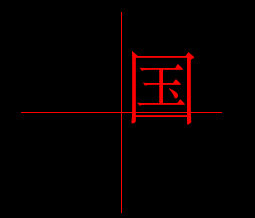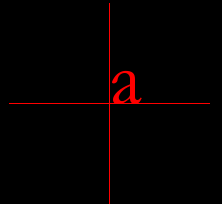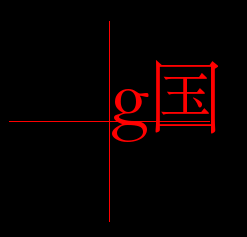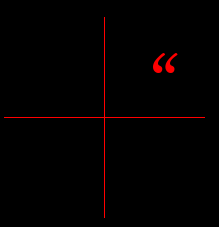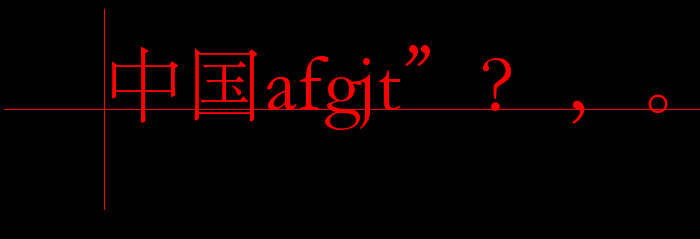（6）\$color 字体的颜色，不多解释。

（7）\$fontfile 字体文件。也就是包含trueType字体字模的文件，如楷体字体文件simkai.ttf。这种文件的格式是有标准规范的，而且与平台无关。所以可以直接把Windows系统的字体文件拷贝到Linux下使用。

（8）\$text 要渲染的字符串。需要注意必须是UTF-8编码的字符串。说到字符串不得不提PHP的string数据类型。虽然名为string，其实PHP语言本身并不认识各种字符编码，它只是简单的把string看做是动态增长的“字节”数组，例如strlen()就是返回的字节数。而我们知道除了ASCII编码的字符和字节是相同的外，几乎没有其他字符编码中的字符对应一个字节，例如一个汉字的UTF-8编码占用3个字节。至于怎么解释其中的字符编码，需要专门的库函数如iconv_strlen()。如果字符串使用字面量，那么其所在的php源文件就必须编码为UTF-8存储。

（1）字处理软件的复杂之处

（2）如何显示加粗字体